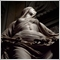# How to open just 1 stop buy/sell order for each 24 hours?21

Hello there,

My strategy consists in sending a stop buy order just at the 00.00 (GMT(0)) hourly candle 2 pips above the previous high, and another stop sell order 2 pips below the previous low. I want these two orders to be sent just once for each 24 hours, starting at 00:00 (GMT(0)). At first it seemed easy to code, but I haven't figured out how to do it yet. Here's the code I've written so far:

```xtern double LotSize = 0.1;
extern int Start_Time = 1;
extern int Finish_Time = 2;
input int Magic = 0;//Magic Number
input int Slip = 3; //Slippage
input int StopRange=10; //Pips to place stop order
input int TP=20; //Pips target
input int SL=20; //Pips stoploss

int ticket, Dgt;
double position, target, stop;
double newlot;

double Pips()

{

// If there are 3 or less digits (JPY for example) then return 0.01 which is the pip value

if(Digits<=3)
{
return(0.01);
}
//If there are 4 or more digits then return 0.0001 which is the pip value
else if(Digits>=4)
{
return(0.0001);
}
//In all other cases (there shouldn't be any) return 0
else return(0);
}

int OnInit()
{

return(INIT_SUCCEEDED);

}

void OnTick()

{

if (TotalOrderCount() == 0 && FoundRecentOpenedOrder(1440) == false)

{

Hour1 = TimeHour(Time);
if(Hour1 == Start_Time) Entry();

}
else
{}

}

int TotalOrderCount(){

int count=0;
for(int pos = OrdersTotal()-1; pos >= 0 ; pos--) if (
OrderSelect(pos, SELECT_BY_POS)                 // Only my orders w/
&&  OrderMagicNumber()  == Magic           // my magic number
&&  OrderSymbol()       == Symbol() ){              // and my pair.
count++;
}
return(count);
}```

The Entry() sets the necessary conditions for the orders to be placed. Any help would be appreciated.5

Nephelion:

Hello there,

My strategy consists in sending a stop buy order just at the 00.00 (GMT(0)) hourly candle 2 pips above the previous high, and another stop sell order 2 pips below the previous low. I want these two orders to be sent just once for each 24 hours, starting at 00:00 (GMT(0)). At first it seemed easy to code, but I haven't figured out how to do it yet. Here's the code I've written so far:

The Entry() sets the necessary conditions for the orders to be placed. Any help would be appreciated.